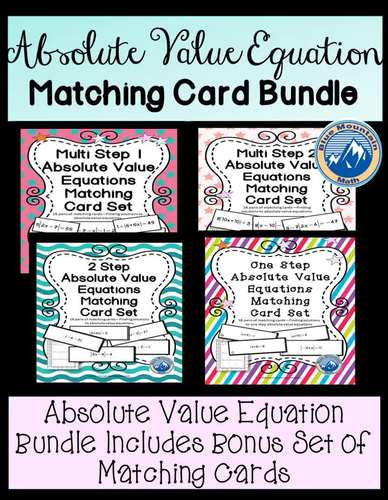# Absolute Value Equation Matching Card BundleSubject
Resource Type
File Type

Zip

(5 MB|57 pages)
Standards
4 Products in this Bundle
4 products
1. Students practice solving absolute value equations in this matching activity to use in a variety of ways. The set includes 18 cards, half the cards have a one variable absolute value equation and the other half of the cards have the solutions. These equations have one operation inside the absolute
2. Students practice solving absolute value equations in this matching activity to use in a variety of ways. The set includes 18 cards, half the cards have a one variable absolute value equation and the other half of the cards have the solutions. These equations have two operations inside the absolut
3. Students practice solving absolute value equations in this matching activity to use in a variety of ways. The set includes 18 cards, half the cards have a one variable absolute value equation and the other half of the cards have the solutions. These equations have one or two operations inside the
4. Students practice solving absolute value equations in this matching activity to use in a variety of ways. The set includes 18 cards, half the cards have a one variable absolute value equation and the other half of the cards have the solutions. These equations have one or two operations inside the
• Bundle Description
• Standards
\$\$\$ Save with the Bundle which Includes a Bonus Matching Card Set

Students practice solving absolute value equations in this matching activity bundle to use in a variety of ways. The set includes 4 sets of 18 pairs of cards, half the cards have an absolute value equation and the other half of the cards have the solutions. Sets include one step absolute value equations, two stes and 2 sets of Multi Step. These equations have one or two operations inside the absolute value bars and zero, one or two operations outside. Suitable for review in Algebra class ,Math 8 and great for test prep

✂️Bonus Set includes 18 pairs of matching cards which mix the absolute value equation types in one set. Bonus Set not available separately.

Cut apart and use for a single student or pair of students.You can use to pair up students for other activities. You can also use these cards as a ticket in the door or ticket out the door. You can prepare ahead of time by printing and laminating for repeated use, or print the sets for each class and allow students to make posters of matching pairs.

Ways to Use Matching Cards.

To see individual sets included here, click on the links:

❤️ Absolute Value One Step Equations Matching Card Set
❤️Absolute Value Two Step Equations Matching Card Set
❤️Absolute Value Multi Step Equation 1 Matching Card Set
❤️ Absolute Value Multi Step Equations Matching Card Set Pt. 2

Create equations and inequalities in one variable and use them to solve problems.
Total Pages
57 pages
Included
Teaching Duration
1 Week
Report this Resource to TpT
Reported resources will be reviewed by our team. Report this resource to let us know if this resource violates TpT’s content guidelines.